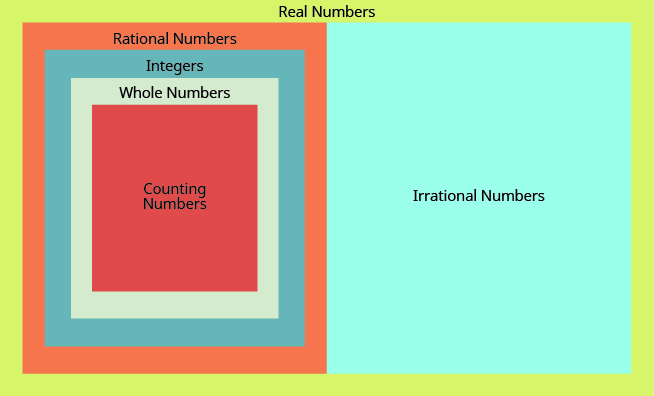## Key Concepts

• Real numbers### The Order of Operations

• Perform all operations within grouping symbols first. Grouping symbols include parentheses ( ), brackets [ ], braces { }, and fraction bars.
• Evaluate exponents or square roots.
• Multiply or divide, from left to right.
• Add or subtract, from left to right.

This order of operations is true for all real numbers.

## Glossary:

Irrational number
A number that cannot be written as the ratio of two integers. Its decimal form does not stop and does not repeat.
Rational number
A number that can be written in the form ${\Large\frac{p}{q}}$ , where p and q are integers and $q\ne 0$ . Its decimal form stops or repeats.
Real number:
A number that is either rational or irrational.

## Contribute!

Did you have an idea for improving this content? We’d love your input.